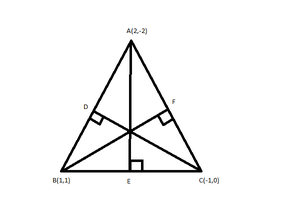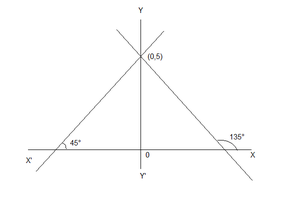GeeksforGeeks App
Open AppBrowser
Continue

## Related Articles

• RD Sharma Class 11 Solutions for Maths

# Class 11 RD Sharma Solutions – Chapter 23 The Straight Lines – Exercise 23.4

### Question 1. Find the equation of straight line passing through the point (6, 2) and having slope -3.

Solution:

We know (y – y1) = m(x – x1)

Here, m = Slope of line = -3

x1 = 6, y1 = 2

So, the equation of line is

⇒ y – 2 = (-3)(x – 6)

⇒ y – 2 = -3x + 18

⇒ 3x + y – 20 = 0

### Question 2. Find the equation of straight line passing through (-2, 3) and inclined at an angle of 45° with the x-axis.

Solution:

We know y – y1 = m(x – x1)

Here, m = Slope of line = tan 45° = 1

x1 = -2, y1 = 3

So, the equation of line is

⇒ y – 3 = 1(x – (-2))

⇒ y – 3 = x + 2

⇒ x – y + 5 = 0

### Question 3. Find the equation of straight line which divides the join of points (2, 3) and (-5, 8) in the ratio 3:4 and is also perpendicular to it.

Solution:

Let the point at which the line divides the join of points (2, 3) and (-5, 8) in the ratio 3:4 be P(x1, y1)

P(x1, y1) = \frac{(4 × 2 - 5 × 3)}{(3 + 4)}, \frac{(4 × 3 + 3 × 8)}{(3+4)}

= (-1, 36/7)

Slope of the given points=(8-3)/(-5-2) =-5/7

Since, the required line is perpendicular to the line joining the given points.

Therefore, the slope of required line ‘m’ = 7/5

Here, x1 = -1, y1 = 36/7 & m = 7/5

So, the equation of line is

⇒ y – 36/7 = 7/5(x – (-1))

⇒ y-36/7 = 7/5(x + 1)

⇒ 35y – 180 = 49x + 49

⇒ 49x + 35y + 229 = 0 is the required equation of straight line.

### Question 4. Prove that the perpendicular drawn from the point (4, 1) on the join of (2, -1) and (6, 5) divides it in ratio 5:8.

Solution:

Let PO be the perpendicular drawn from P(4,1) on the line joining A(2, -1) and B(6, 5)

Let slope of PO be ‘m’

According to question

m × Slope of AB = -1

⇒ m × (5 + 1)/(6 – 2) = -1

⇒ m × 6/4 = -1

⇒ m = -4/6 = -2/3

Thus, the equation of line PO

x1 = 4, y1 = 1 & m = -2/3

(y – 1) = -2/3(x – 4)

⇒ 3y – 3 = -2x + 8

⇒ 2x + 3y – 11 = 0 ——–(1)

Let O divide the line AB in the ratio of K:1

Then the coordinates of O are \frac{(6k + 2)}{(k + 1)}, \frac{(5k - 1)}{(k + 1)}

Since point O lies in the line AB

Therefore, it satisfies the equation (1)⇒ 12k + 4 + 15k – 3 – 11(k + 1) = 0

⇒ 27k – 11k + 1 – 11 = 0

⇒ 16k = 10

⇒ k = 5/8

Hence Proved

### Question 5. Find the equation to the altitudes of the triangle whose angular points are A(2, -2), B(1, 1), and C(-1, 0).

Solution:

Let the altitudes be AE, BF and CDSlope of AE × Slope of BC = -1                       [Since both lines are perpendicular to each other]

Slope of AE = (-1) / Slope of BC

Slope of AE == -2

Equation of the altitude AE

y – (-2) = (-2)(x – 2)

⇒ y + 2 = -2x + 4

⇒ 2x + y – 2 = 0

Similarly,

Slope of BF × Slope of AC = -1

Slope of BF == 3/2

Equation of the altitude BF

y – 1 = (3/2)(x – 1)

⇒ 2(y – 1) = 3x – 3

⇒ -3x + 2y – 2 + 3 = 0

⇒ 3x – 2y – 1 = 0

Slope of CD × Slope of AB = -1

Slope of CD== 1/3

Equation of the altitude CD

y – 0 = (1/3) (x – (-1))

⇒ 3y = x + 1

⇒ x – 3y + 1 = 0

### Question 6. Find the equation of the right bisector of the line segment joining the points (3, 4) and (-1, 2).

Solution:

Let the points of the line segment be A(3, 4) and B(-1, 2)

Let the right bisector meet at point ‘P’ on the line segment

Coordinates of point P = \frac{(3-1)}{2}, \frac{(4+2)}{2}          [Using the mid point formula]

= (1, 3)

Slope of AB = [(2 – 4) / (-1 – 3)]

=-2/-4

= 1/2

Slope of right bisector == -2

Equation of the right bisector

⇒ y – 3 = -2(x – 1)

⇒ y – 3 = -2x + 2

⇒ 2x + y – 5 = 0 is the required equation of the right bisector

### Question 7. Find the equation of the straight line passing through the point (3, -2) and making an angle of 60° with the positive direction y-axis.

Solution:

Since the line is making an angle of 60° with the positive direction of y-axis i

t makes an angle of 30° with the positive direction of x-axis as shown in the diagram.

Slope of line ‘m’= tan 30° = 1/√3

Equation of straight line passing through (3, -2)

y – (-2) = 1/√3(x – 3)

⇒ √3(y + 2) = x – 3

⇒ √3y + 2√3 = x – 3

x – √3y – 3 – 2√3 = 0 is the required equation of straight line.

### Question 8. Find the equation of the straight line which passes through the point (1, 2) and makes an angle with the positive direction of x-axis whose sine is 3/5.

Solution:

Given, sin θ = 3/5

tan θ = 3/√(25 – 9) = 3/4

Slope of the line m = 3/4

Equation of straight line passing through (1, 2)

y – 2 = 3/4(x – 1)

⇒ 4(y – 2) = 3x – 3

⇒ 4y – 8 = 3x – 3

⇒ 3x – 4y + 5 = 0 is the required equation of straight line.

### Question 9. Find the equation of the line passing through the point (-3, 5) and perpendicular to the line joining (2, 5) and (-3, 6).

Solution:

Slope of the required equation ‘m’ = (-1) / [Slope of line joining (2, 5) and (-3, 6)]                 [Perpendicular to each other]

m =m =m = 5

Equation of straight line passing through (-3, 5)

y – 5 = 5(x – (-3))

⇒ y – 5 = 5x + 15

⇒ 5x – y + 20 = 0 is the required equation of straight line.

### Question 10. Find the equation of the right bisector of the line segment joining the points A(1, 0) and B(2, 3).

Solution:

Let the right bisector meet at point ‘P’ on the line segment

Coordinates of point P = []                   [Using the mid point formula]

= (3/2, 3/2)

Slope of AB = [(3 – 0)/(2 – 1)]

= 3/1 = 3

Slope of right bisector = -1/3

Equation of the right bisector

⇒ y – 3/2 = -1/3(x – 3/2)

⇒ 3(y – 3/2) = -x + 3/2

⇒ x + 3y – 9/2 – 3/2 = 0

⇒ x + 3y – 6 = 0

x + 3y – 6 = 0 is the required equation of  the right bisector.

### Question 11. Find the lines through the point (0, 2) making angles π/3 and 2π/3 with the x-axis. Also, find the lines parallel to them cutting the y-axis at a distance of 2 units below the origin.

Solution:

Given the line through the point (0, 2) making angles π/3 and 2π/3 with the x-axis

.Slope m1= tan π/3 = √3

Slope m2 = tan 2π/3 = -√3

Equation of the required lines

⇒ y – 2 = √3(x – 0) and y – 2 = -√3(x – 0)

⇒ y – √3x – 2 = 0 and y + √3x – 2 = 0

Now, the equation of the line parallel to the line having slope m1 and y intercept c= -2

y = m1x + c

⇒ y = √3x – 2

Similarly, the equation of the line parallel to the line having slope m2 and y intercept c = -2

y= m2x + c

⇒ y = -√3x – 2

### Question 12.  Find the equation of the straight lines which cut off an intercept 5 from the y-axis and are equally inclined to the axes.

Solution:

Given that the straight lines cut off an intercept 5 from the y-axis and are equally inclined to the axes.Slope of the two lines are m1= tan 45° =1 and m2 = tan 135° = -1

Equation of the required straight lines are

y = m1x + c or y = m2 + c

⇒ y = x +5 or y = -x + 5

⇒ y = x +5 or y + x = 5

### Question 13.  Find the equation of straight lines which intercepts a length 2 on the positive direction of the x-axis and is inclined at an angle of 135° with the positive direction of y-axis.

Solution:

The required line which is inclined at an angle of 135° with the positive direction of y-axis makes an angle of 45° with the positive x-axis.

Slope of the required line m = tan 45° = -1

Equation of the required straight line with x-intercept c = 2 and m = -1

x = my + c

⇒ x = 1y + 2

⇒ x – y  – 2 = 0

### Question 14. Find the equation of line passing through (0, 0) with slope m.

Solution:

Equation of line passing through (0, 0) with slope m is

y – 0 = m(x – 0)

y = mx

### Question 15. Find the equation of line passing through (2, 2√3) and inclined with x-axis at an angle of 75°.

Solution:

Slope of the line m = tan 75°

= tan (45° + 30°)

= (tan 45° + tan30°) /(1 – tan 45° tan30°)

= (1 + 1/√3)/(1 – 1/√3)

m = (√3 + 1)/(√3 – 1) = 2 + √3

Equation of the required line passing through (2, 2√3) with slope of 2 + √3

y – 2√3 = (2 + √3)(x – 2)

⇒ y – 2√3  = (2 + √3)x – 4 – 2√3

(2 + √3)x – y – 4 = 0

### Question 16. Find the equation of the line passing through(1, 2) and making angle of 30° with the y-axis.

Solution:

Let us considered an equation of line passing through points(x1, y1) which making an angle θ with x-axis.

(y – y1) = tanθ(x – x1)         -(1)

Given: Point = (1, 2), and angle = 30°(with y-axis)

So, angle with x-axis = 90° – 30° = 60°

Now put all these values in eq(1), we get

(y – 2) = tan60°(x – 1)

(y – 2) = √3(x – 1)

y – 2 = √3x – √3

√3x – √3 – y + 2 = 0

√3x – y – √3  + 2 = 0

My Personal Notes arrow_drop_up
Related Tutorials